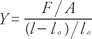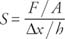## Elasticity and Simple Harmonic Motion

A rigid body is an idealization because even the strongest material deforms slightly when a force is applied. Elasticity is the field of physics that studies the relationships between solid body deformations and the forces that cause them.

## Elastic modules

In general, an elastic modulus is the ratio of stress to strain. Young's modulus, the bulk modulus, and the shear modulus describe the response of an object when subjected to tensile, compressional, and shear stresses, respectively. When an object such as a wire or a rod is subjected to a tension, the object's length increases. Young's modulus is defined as the ratio of tensile stress and tensile strain. Tensile stress is a measure of the deformation that causes stress. Its definition is the ratio of tensile force (F) and the cross‐sectional area normal to the direction of the force (A). Units of stress are newtons per square meter (N/m 2). Tensile strain is defined as the ratio of the change in length ( l ol) to the original length ( l o). Strain is a number without units; therefore, the expression for Young's modulus isIf an object of cubic shape has a force applied pushing each face inward, a compressional stress occurs. Pressure is defined as force per area P = F/A. The SI unit of pressure is the pascal, which is equal to 1 newton/meter 2 or N/m 2. Under uniform pressure, the object will contract, and its fractional change in volume (V) is the compressional strain. The corresponding elastic modulus is called the bulk modulus and is given by B = − P/(Δ V/ V o). The negative sign ensures that B is always a positive number because an increase in pressure causes a decrease in volume.

Applying a force on the top of an object that is parallel to the surface on which it rests causes a deformation. For example, push the top of a book resting on a tabletop so that the force is parallel to surface. The cross‐section shape will change from a rectangle to a parallelogram due to the shear stress (see Figure 1). Shear stress is defined as the ratio of the tangential force to the area (A) of the face being stressed. Shear strain is the ratio of the horizontal distance the sheared face moves (Δ x) and the height of the object (h), which leads to the shear modulus:Figure 1

Shear stress deforms a book.

## Hooke's Law

The direct relationship between an applied force and the change in length of a spring, called Hooke's law, is F = − kx, where x is the stretch in the spring and k is defined as the spring constant. Units for k are newtons per meter. When a mass is hung on the end of the spring, at equilibrium the downward gravitational force on the mass must be balanced by an upward force due to the spring. This force is called the restoring force. The negative sign indicates that the direction of the restoring force due to the spring is in the opposite direction from the stretch, or displacement, of the spring.

## Simple harmonic motion

A mass bouncing up and down on the end of a spring undergoes vibrational motion. The motion of any system whose acceleration is proportional to the negative of displacement is termed simple harmonic motion (SHM), i.e. F = ma = −kx. Certain definitions pertain to SHM:

• A complete vibration is one down and up motion.
• The time for one complete vibration is the period, measured in seconds.
• The frequency is the number of complete vibrations per second and is defined as the reciprocal of the period. Its units are cycles/second or hertz (Hz).
• The amplitude is the absolute value of the distance from the maximum vertical displacement to the central point of the motion, that is, the greatest distance up or down the mass moves from its initial position.

The equation relating to period, the mass, and the spring constant is T = 2π√ m/ k. This relationship gives the period in seconds.

## The relation of SHM to circular motion

Aspects of SHM can be visualized by looking at its relationship to uniform circular motion. Imagine a pencil taped vertically to a horizontal turntable. View the rotating pencil from the side of the turntable. As the turntable rotates with uniform circular motion, the pencil moves back and forth with simple harmonic motion. Figure (a) illustrates P as the point on the rim of the turntable—the position of the pencil. Point P′ indicates the apparent position of the pencil when viewing only the x component. The acceleration vector and vector components are shown in Figure 2(b).Figure 2

The relationship between circular motion and SHM.

The following is proof of the relationship between SHM and one component of uniform circular motion. This component of motion is that observed by looking at circular motion from the side. The maximum displacement of the component of the uniform circular motion is the radius of the circle (A). Substitute the radius of the circle (A) into the equations for angular velocity and angular acceleration to obtain v = rω = Aω and a = v 2/ r = rω 2 = Aω 2. The horizontal component of this acceleration is a = − Aω o sin θ = −ω 2 x, using x = A as shown in Figure . Because the acceleration is proportional to the displacement, the point rotating with uniform circular motion undergoes SHM when only one component of the motion is considered.

## The simple pendulum

The simple pendulum is the idealized model of a mass swinging on the end of a massless string. For small arcs of swing of less than 15 degrees, the motion of the pendulum approximates SHM. The period of the pendulum is given by T = 2π√ l/ g, where l is the length of the pendulum and g is the acceleration due to gravity. Notice that the period of a pendulum is not dependent upon the pendulum's mass.

## SHM energy

The potential energy of a Hooke's law spring is P. E.=(1/2) kx 2. The total energy is the sum of the kinetic and potential energies at any time and is conserved.